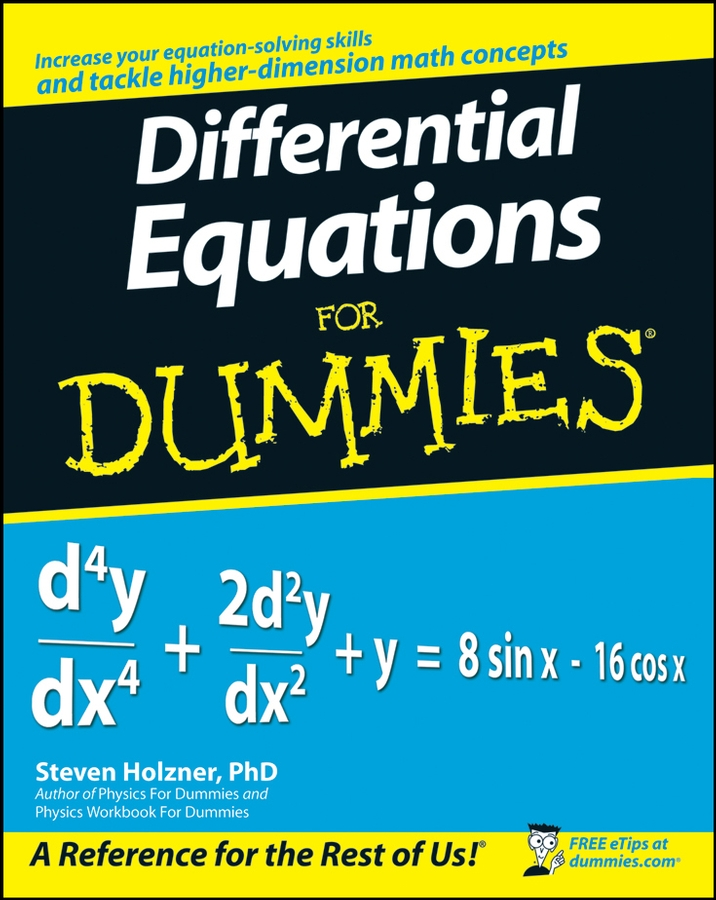##### Differential Equations For DummiesIn calculus, the way you solve a derivative problem depends on what form the problem takes. Common problem types include the chain rule; optimization; position, velocity, and acceleration; and related rates. Here are a few things to remember when solving each type of problem:

### Chain Rule problems

1. Use the chain rule when the argument of the function you’re differentiating is more than a plain old x.

2. Work from outside, in.

3. Don’t touch the inside stuff.

4. Do only one derivative per step.

### Optimization problems

1. Express the thing you want to minimize or maximize as a function of the unknown.

2. Differentiate and set the derivative equal to zero.

3. Solve and plug the solution into the original function.

### Position, velocity, and acceleration problems

1. The derivative of position is velocity and the antiderivative of velocity is position.

2. The derivative of velocity is acceleration and the antiderivative of acceleration is velocity.

### Related rates problems

1. Assign variables to changing quantities, but not to unchanging things.

2. Differentiate before plugging in variable values.

3. Use the Pythagorean Theorem for right triangle problems and use similar triangles for problems involving cones or shapes that have a triangular cross-section.